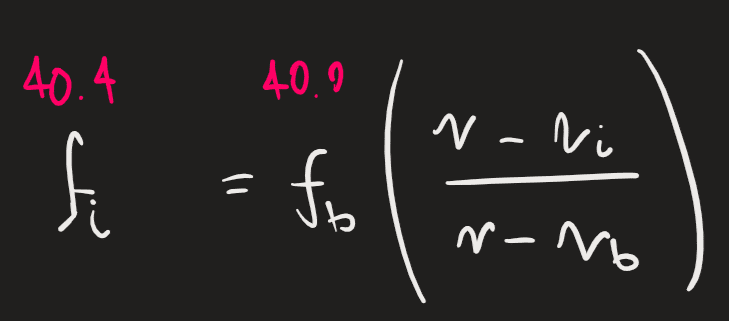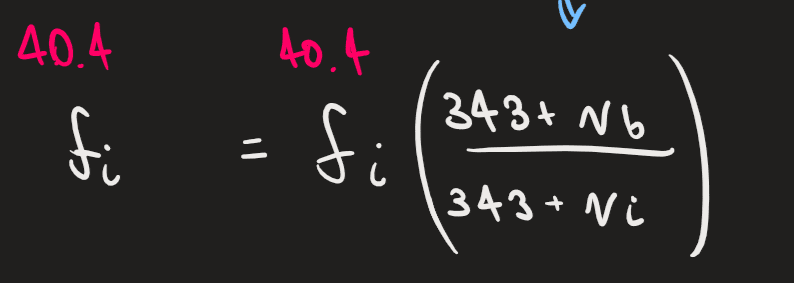# Doppler Effect -- Bat Problem

• physicsfan999
In summary, the problem involves the Doppler effect with a bat chasing an insect at 4.10 m/s. The bat emits a 40.0-kHz chirp and receives back an echo at 40.4 kHz from the insect. To solve this, we treat the bat as the source in the first step and the observer in the second step. The final equation should have a different symbol for the observed frequency by the bat.f

#### physicsfan999

Homework Statement
A bat flying at 4.10 m/s is chasing an insect flying in the same direction. The bat emits a 40.0-kHz chirp and receives back an echo at 40.4 kHz. (Take the speed of sound in air to be 340 m/s.)
Relevant Equations
f_o=f_s(v+v_o/v-v_s)
I'm struggling a lot with this problem on the Doppler effect. I understand the first step which is to treat the bat as the source of the emitted sound, givingAnd the second to treat the bat now as the observer, but instead of using f_b on the left the solution involves setting both frequencies to the reflected wave.I understand there should 2 different variables here for the equation to make sense but I need help understanding why the second step involves setting the two frequencies the same. Thanks in advance!

Homework Statement:: A bat flying at 4.10 m/s is chasing an insect flying in the same direction. The bat emits a 40.0-kHz chirp and receives back an echo at 40.4 kHz. (Take the speed of sound in air to be 340 m/s.)
Relevant Equations:: f_o=f_s(v+v_o/v-v_s)

I'm struggling a lot with this problem on the Doppler effect. I understand the first step which is to treat the bat as the source of the emitted sound, giving

View attachment 279012

And the second to treat the bat now as the observer, but instead of using f_b on the left the solution involves setting both frequencies to the reflected wave.
View attachment 279011
I understand there should 2 different variables here for the equation to make sense but I need help understanding why the second step involves setting the two frequencies the same. Thanks in advance!
As you correctly say, this is a 2-stage process. In stage-1 we find the observed frequency (##f_i##) with the insect as the observer). In stage-2 we treat ##f_i## as the source frequency because this is the frequency of the reflected signal sent from the insect to the bat.

Your final equation$$f_i = f_i (\frac {343 + v_b}{343 + v_i})$$is wrong. You need to give the final observed frequency (by the bat) a different symbol:$$f_{observed-by-bat} = f_i (\frac {343 + v_b}{343 + v_i})$$Can I suggest you watch this:
Edit - typo' corrected.

•collinsmark, Doc Al and rsk